JEE  >  Competition Level Test: Relations And Functions- 2

# Competition Level Test: Relations And Functions- 2

Test Description

## 30 Questions MCQ Test JEE Foundation | Competition Level Test: Relations And Functions- 2

Competition Level Test: Relations And Functions- 2 for JEE 2023 is part of JEE Foundation preparation. The Competition Level Test: Relations And Functions- 2 questions and answers have been prepared according to the JEE exam syllabus.The Competition Level Test: Relations And Functions- 2 MCQs are made for JEE 2023 Exam. Find important definitions, questions, notes, meanings, examples, exercises, MCQs and online tests for Competition Level Test: Relations And Functions- 2 below.
Solutions of Competition Level Test: Relations And Functions- 2 questions in English are available as part of our JEE Foundation for JEE & Competition Level Test: Relations And Functions- 2 solutions in Hindi for JEE Foundation course. Download more important topics, notes, lectures and mock test series for JEE Exam by signing up for free. Attempt Competition Level Test: Relations And Functions- 2 | 30 questions in 60 minutes | Mock test for JEE preparation | Free important questions MCQ to study JEE Foundation for JEE Exam | Download free PDF with solutions
 1 Crore+ students have signed up on EduRev. Have you?
Competition Level Test: Relations And Functions- 2 - Question 1

### Let  A = {1, 2, 3} and let R = {(1, 1), (2, 2), (3, 3), (1, 3), (3, 2), (1, 2)}. Then R is

Detailed Solution for Competition Level Test: Relations And Functions- 2 - Question 1

R is reflexive and transitive but not symmetric

Competition Level Test: Relations And Functions- 2 - Question 2

### Let A {a, b, c} and let R = {(a, a)(a, b), (b, a)}. Then, R is

Detailed Solution for Competition Level Test: Relations And Functions- 2 - Question 2

R is symmetric and transitive but not reflexive

Competition Level Test: Relations And Functions- 2 - Question 3

### Let A = {1, 2, 3} then total number of element in A x A is

Competition Level Test: Relations And Functions- 2 - Question 4

Let S be the set of all straight lines in a plane. Let R be a relation on S defined by a R b ⇔ a ⊥ b. then, R is

Detailed Solution for Competition Level Test: Relations And Functions- 2 - Question 4

a ⊥ a is not true. So, R is not reflexive
a ⊥ b and b ⊥ c does not imply a ⊥ c. So, R is not transitive
But, a ⊥ b ⇒ b ⊥ a is always true.

Competition Level Test: Relations And Functions- 2 - Question 5

Let S be the set of all real numbers and let R be a relation on S, defined by a R b ⇔ |a – b| < 1. Then, R is

Detailed Solution for Competition Level Test: Relations And Functions- 2 - Question 5

(i) |a – a| = 0 < 1 is always true
(ii) a R b ⇒ |a – b| < 1 ⇒ |-(a – b)| < ⇒ |b – a| < 1 ⇒ b R a.
(iii) 2R 1 and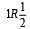But, 2 is not related to 1/2. So, R is not transitive.

Competition Level Test: Relations And Functions- 2 - Question 6

Domain of f (x)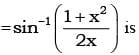Detailed Solution for Competition Level Test: Relations And Functions- 2 - Question 6

Domain of sin -1x is [-1,1]

Competition Level Test: Relations And Functions- 2 - Question 7

Let R be the relation in the set N given by R = {(a, b): a = b – 2, b > 6}. Choose the correct answer.

Detailed Solution for Competition Level Test: Relations And Functions- 2 - Question 7

R = {(a, b): a = b − 2, b > 6}
Now, since b > 6, (2, 4) ∉ R
Also, as 3 ≠ 8 − 2, (3, 8) ∉ R
And, as 8 ≠ 7 − 2
∴ (8, 7) ∉ R
Now, consider (6, 8).
We have 8 > 6 and also, 6 = 8 − 2.
∴ (6, 8) ∈ R

Competition Level Test: Relations And Functions- 2 - Question 8

Let R = {(3, 3), (6, 6), (9, 9), (3,6), (3, 9), (9, 12), (3,12), (6, 12), (12, 12)}, be a relation on the set A = {3, 6, 9, 12} Then the relation is

Detailed Solution for Competition Level Test: Relations And Functions- 2 - Question 8

R is reflexive
∴ (3, 3), (6,6), (9, 9), (12, 12) ∈ R
again ∴ (6, 12) ∈ R but (12, 6) ∉ R ⇒ R is not symmetric
R is transitive
[∴ (3, 6) ∈ R, (6, 12) ∈ R, (3, 12) ∈ R others are clear

Competition Level Test: Relations And Functions- 2 - Question 9

If f(x) = (a – xn)1/n.  then f(f(x)) =

Detailed Solution for Competition Level Test: Relations And Functions- 2 - Question 9

f(x) = (a – xn)1/n = y

Competition Level Test: Relations And Functions- 2 - Question 10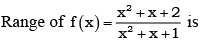Competition Level Test: Relations And Functions- 2 - Question 11

f: N → N : f(x) = 2x is

Detailed Solution for Competition Level Test: Relations And Functions- 2 - Question 11

The function is injective (one-to-one) if every element of the codomain is mapped to by at most one element of the domain

The function is surjective (onto) if every element of the codomain is mapped to by at least one element of the domain

f(x)=2x

Domain of f is N

Codomain of f is N

Every element of N(codomain) is mapped to only one element in N(domain)

i.e. f(x)=f(y)

⟹2x=2y⟹x=y

Hence f is one one function

Every even element of N(codomain) has corresponding value in N(domain).

But, for any odd number in N(codomain) has no corresponding value in Ndomain.

Hence, f is not onto function.

Competition Level Test: Relations And Functions- 2 - Question 12

f: R → R : f(x) = x2 is

Detailed Solution for Competition Level Test: Relations And Functions- 2 - Question 12

Given, function f : R→R such that f(x) = x2
Let A and B be two sets of real numbers.
Let x1, x2 ∈ A such that f(x1) = f(x2).
⇒1 + (x1)2 = 1 + (x2)2
​⇒ (x1)2 − (x2)2 = 0
⇒(x1 − x2)(x1 + x2)=0
⇒ x1 = ± x2
Thus f(x1) = f(x2) does not imply that x1 = x2.
For instance, f(1) = f(−1) = 1, i.e. , two elements (1, -1) of A have the same image in B. So, f is many-one function.

Competition Level Test: Relations And Functions- 2 - Question 13

f : R → R : f(x) = x3 is

Detailed Solution for Competition Level Test: Relations And Functions- 2 - Question 13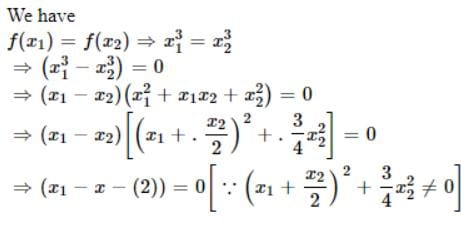⇒ x2 = x2

∴ f is one-one

Let y∈R and let y=x3. then x=y ∈ R

Thus for each y in the codomain R there exists y1/3 in R such that

f(y13)=(y13)3=y

∴ f is onto.

Hence f is one -one onto.

Competition Level Test: Relations And Functions- 2 - Question 14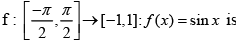Detailed Solution for Competition Level Test: Relations And Functions- 2 - Question 14

f(x) = sinx
f(x) = sinx is a one-one function.
codomain = range
therefore, f(x) = sinx is an onto function.

Competition Level Test: Relations And Functions- 2 - Question 15

f : R → R : f(x) = cos x is

Detailed Solution for Competition Level Test: Relations And Functions- 2 - Question 15

cos(2π - θ) = cosq ⇒ f is many-one.
Range (f) = [-1,1] ⊂ R ⇒ f is in to.

Competition Level Test: Relations And Functions- 2 - Question 16

The domain of the function f = {(1, 3), (3, 5), (2, 6)} is

Detailed Solution for Competition Level Test: Relations And Functions- 2 - Question 16

Domain = {1, 3, 2}

Competition Level Test: Relations And Functions- 2 - Question 17

Let f(x) = x - 1/x +1 , x ≠ -1, then f-1 (x) is

Competition Level Test: Relations And Functions- 2 - Question 18

If f(x) = x/x -1 , x ≠ 1, then f-1 (x) is

Competition Level Test: Relations And Functions- 2 - Question 19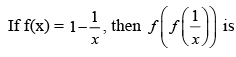Competition Level Test: Relations And Functions- 2 - Question 20

If f(x) = cos (log x), then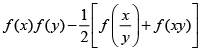has the value

Detailed Solution for Competition Level Test: Relations And Functions- 2 - Question 20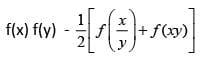= cos (log x) cos (cos y)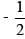[cos(log(x/y))+cos(log(xy))]
= cos (log x) cos (log y)[cos(log x - log y)+cos(log x +log y)]
= cos (log x) cos (log y)[2 cos(log  x)cos(log y)] = 0

Competition Level Test: Relations And Functions- 2 - Question 21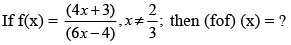Detailed Solution for Competition Level Test: Relations And Functions- 2 - Question 21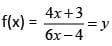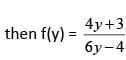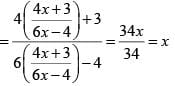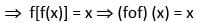Competition Level Test: Relations And Functions- 2 - Question 22

If f(x) =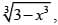then (fof) (x) = ?

Detailed Solution for Competition Level Test: Relations And Functions- 2 - Question 22

(fof) (x) = f[f(x)] – {(3 – x3)1/3} = f(y)  where y = (3 – x3)1/3
= (3 – y3)1/3 = [3- (3 – x3)]1/3 = (x3)1/3 = x

Competition Level Test: Relations And Functions- 2 - Question 23

If f(x) = x2 – 3x + 2, then (fof) (x) = ?

Detailed Solution for Competition Level Test: Relations And Functions- 2 - Question 23

(fof) (x) = f[f(x)] = f(x2 - 3x + 2)2 - 3(x2 – 3x + 2)
= y2 – 3y + 2 = (x2 – 3x + 2)2 – 3(x2 – 3x + 2) + 2 =  (x4 – 6x3 + 10x2 – 3x)

Competition Level Test: Relations And Functions- 2 - Question 24

Let S = {1, 2, 3}. The function f : S → S defined as below have inverse for

Detailed Solution for Competition Level Test: Relations And Functions- 2 - Question 24

Since f(2) = f(3) = 1, then inverse does not exists
Inverse exist for (C) f-1 = {(3, 1), (2, 3), (1, 2)}.

Competition Level Test: Relations And Functions- 2 - Question 25

The relation R defined on the set N of natural numbers by xRy ⇔2x2 - 3xy + y2 = 0 is

Detailed Solution for Competition Level Test: Relations And Functions- 2 - Question 25

(i) xRx ⇔ 2x2 - 3x.x + x2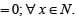∴ R is reflexive
(ii) For x = 1, y= 2; 2x2 - 3xy + y2 = 0
∴ 1 R 2 but 2.22 - 3.2.1 + 12 = 3 ≠ 0.
So, 2 is not R-related to 1.
∴ R is not symmetric.

Competition Level Test: Relations And Functions- 2 - Question 26

Let R = {(3, 3), (6, 6), (9, 9), (12, 12), (6, 12), (3, 9), (3, 12), (3, 6) be a relation on the set A = {3, 6, 9, 12}. The relation is

Detailed Solution for Competition Level Test: Relations And Functions- 2 - Question 26

Since, (3, 3), (6, 6), (9, 9), (12, 12) ∈ R ⇒ R is reflexive relation.
Now, (6, 12)∉R but (12, 6) ∉ R  ⇒ R is not a symmetric relation.
Also, (3, 6),(6, 12) ∈ R ⇒ (3, 12) ∈ R
⇒ R is transitive relation.

Competition Level Test: Relations And Functions- 2 - Question 27

Let R be a relation on the set A of ordered pairs of positive integers defined by (x, y)R(u, v) if and only if xv = yu, then

Detailed Solution for Competition Level Test: Relations And Functions- 2 - Question 27

Clearly, (x, y)R(x, y),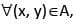since xy = yx. This shows that R is reflexive.
Further, (x, y)R(u, v) ⇒ xv = yu ⇒ uy vx and hence (u, v)R(x, y). This shows that R is symmetric.
Similarly, (x, y)R(u, v) and (u, v)R(a, b) ⇒ xv = yu and ub = va ⇒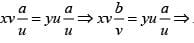xb = ya and hence (x, y)R(a, b). Thus, R is transitive. Thus, R is an equivalent relation.

Competition Level Test: Relations And Functions- 2 - Question 28

If R be a relation defined as aRb iff |a -b|> 0, then the relation is

Detailed Solution for Competition Level Test: Relations And Functions- 2 - Question 28

Since, R is a defined as aRb iff |a – b| > 0
For reflexive aRa iff |a – a| > 0
Which is not true, So, R is not reflexive
For symmetric aRb iff |a – b| > 0
Now bRa iff |b – a| > 0
⇒ |a – b| > 0  ⇒ ArB
Thus, R is symmetric.
For transitive aRb iff |a – b| > 0
bRc iff |b – c| > 0
⇒ |a – b + b – c| > 0
⇒ |a – c| > 0 ⇒ | c – a| > 0 ⇒ aRc
∴ R is transitive

Competition Level Test: Relations And Functions- 2 - Question 29

If R is an equivalence relation of a set A, then R-1 is

Detailed Solution for Competition Level Test: Relations And Functions- 2 - Question 29

If R is an equivalence relation, then R-1 is also an equivalence relation.

Competition Level Test: Relations And Functions- 2 - Question 30

Let r be a relation from R (set of real numbers) to R defined by r = {(a, b)|a, b∈R and a - b + √3 is an irrational number}. The relation r is

Detailed Solution for Competition Level Test: Relations And Functions- 2 - Question 30

Given, r = {(a, b)| a, b∈R and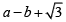as an irrational number
(i) Reflexive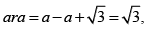which is irrational number.
(ii) Symmetric
Now,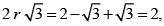which is not an irrational.
Also,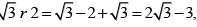which is an irrational.
Which is not symmetric.
(iii) Transitive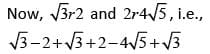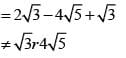∴ It is not transitive.

## JEE Foundation

99 videos|291 docs|212 tests
Information about Competition Level Test: Relations And Functions- 2 Page
In this test you can find the Exam questions for Competition Level Test: Relations And Functions- 2 solved & explained in the simplest way possible. Besides giving Questions and answers for Competition Level Test: Relations And Functions- 2, EduRev gives you an ample number of Online tests for practice

## JEE Foundation

99 videos|291 docs|212 tests(Scan QR code)